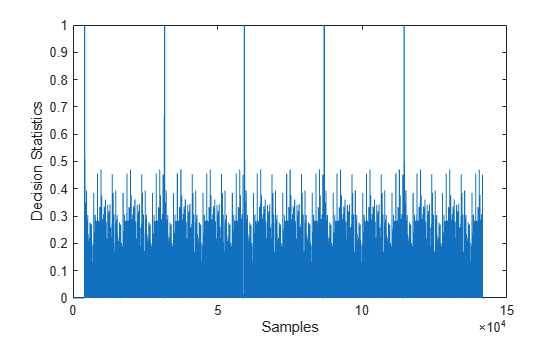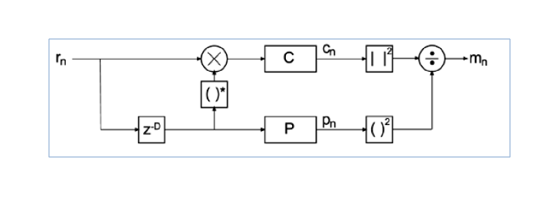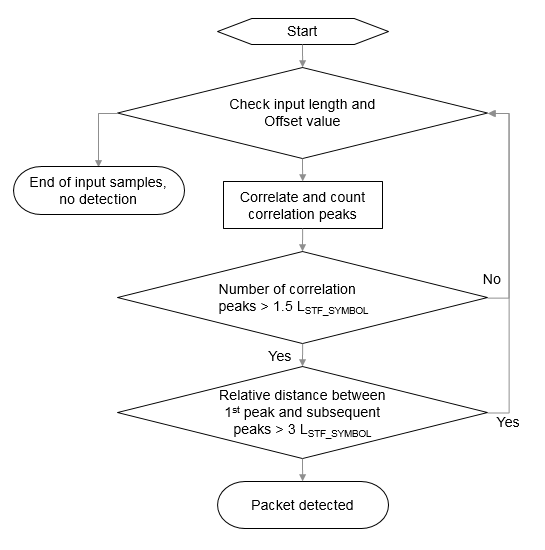# wlanPacketDetect

Estimate timing offset of OFDM packet

## Syntax

``startOffset = wlanPacketDetect(rxSig,cbw)``
``startOffset = wlanPacketDetect(rxSig,cbw,offset)``
``startOffset = wlanPacketDetect(rxSig,cbw,offset,threshold)``
``````[startOffset,M] = wlanPacketDetect(___)``````

## Description

example

````startOffset = wlanPacketDetect(rxSig,cbw)` estimates the timing offset between the start of received signal `rxSig` and the start of the detected preamble for channel bandwidth `cbw`. For more information, see Packet Detection Processing. NoteThis function supports packet detection of OFDM modulated signals only. ```

example

````startOffset = wlanPacketDetect(rxSig,cbw,offset)` specifies the sample at which the function begins autocorrelation processing relative to the start of the received signal.```

example

````startOffset = wlanPacketDetect(rxSig,cbw,offset,threshold)` specifies the threshold that the decision statistic must meet or exceed to detect a packet. ```

example

``````[startOffset,M] = wlanPacketDetect(___)``` also returns the decision statistics of the packet detection algorithm for any of the input argument combinations in previous syntaxes.```

## Examples

collapse all

Detect a received 802.11n™ packet at a signal-to-noise ratio (SNR) of 20 dB.

Create an HT configuration object and TGn channel object. Generate a transmit waveform.

```cfgHT = wlanHTConfig; tgn = wlanTGnChannel('LargeScaleFadingEffect','None'); txWaveform = wlanWaveformGenerator([1;0;0;1],cfgHT);```

Pass the waveform through a TGn channel with an SNR of 20 dB. Detect the start of the packet.

```snr = 20; fadedSig = tgn(txWaveform); rxWaveform = awgn(fadedSig,snr,0); startOffset = wlanPacketDetect(rxWaveform,cfgHT.ChannelBandwidth)```
```startOffset = 1 ```

Detect a received 802.11ac™ packet that has been delayed. Specify an offset of 25 to begin the autocorrelation process.

Create an VHT configuration object and generate the transmit waveform.

```cfgVHT = wlanVHTConfig; txWaveform = wlanWaveformGenerator([1;0;0;1],cfgVHT,... 'WindowTransitionTime',0);```

Delay the signal by appending zeros at the start. Specify an offset of 25 for the beginning of autocorrelation processing. Detect the start of the packet.

```rxWaveform = [zeros(100,1);txWaveform]; offset = 25; startOffset = wlanPacketDetect(rxWaveform,cfgVHT.ChannelBandwidth,offset)```
```startOffset = 48 ```

Calculate the detected packet offset by adding the returned `startOffset` and the input `offset`. This coarse approximation of the packet-start offset is useful for determining where to begin autocorrelation for the first packet and for subsequent packets when a multipacket waveform is transmitted.

`pktOffset = offset + startOffset`
```pktOffset = 73 ```

Detect a received 802.11a™ packet that has been delayed. No channel impairments are added. Set the input offset to 5 and use a threshold setting very close to 1.

Create an non-HT configuration object. Generate the transmit waveform.

```cfgNonHT = wlanNonHTConfig; txWaveform = wlanWaveformGenerator([1;0;0;1],cfgNonHT,... 'WindowTransitionTime',0);```

Delay the signal by appending zeros at the start. Set an initial offset of 5 and a threshold very close to 1. Detect the delayed packet.

```rxWaveform = [zeros(20,1);txWaveform]; offset = 5; threshold = 1-10*eps; startOffset = wlanPacketDetect(rxWaveform,... cfgNonHT.ChannelBandwidth,offset,threshold)```
```startOffset = 15 ```

Calculate the detected packet offset by adding the returned `startOffset` and the input `offset`.

`totalOffset = offset + startOffset`
```totalOffset = 20 ```

Return the decision statistics of a WLAN waveform that consists of five 802.11a packets.

Create a non-HT configuration object and a five-packet waveform. Delay the waveform by 4000 samples.

```cfgNonHT = wlanNonHTConfig; txWaveform = wlanWaveformGenerator([1;0;0;1],cfgNonHT, ... 'NumPackets',5,'IdleTime',20e-6); rxWaveform = [zeros(4000,1);txWaveform];```

Generate and plot packet decision statistics for the waveform. The decision statistics show five peaks, which correspond to the first sample of each packet detected.

```offset = 0; threshold = 1; [startOffset,M] = wlanPacketDetect(rxWaveform,cfgNonHT.ChannelBandwidth,... offset,threshold); plot(M) xlabel('Samples') ylabel('Decision Statistics')```## Input Arguments

collapse all

Received time-domain signal, specified as a complex-valued matrix of size NS-by-NR matrix. NS is the number of time-domain samples in the received signal. NR is the number of receive antennas.

Data Types: `single` | `double`
Complex Number Support: Yes

Channel bandwidth, specified as one of these values.

• `'CBW5'` – Channel bandwidth of 5 MHz

• `'CBW10'` – Channel bandwidth of 10 MHz

• `'CBW20'` – Channel bandwidth of 20 MHz

• `'CBW40'` – Channel bandwidth of 40 MHz

• `'CBW80'` – Channel bandwidth of 80 MHz

• `'CBW160'` – Channel bandwidth of 160 MHz

• `'CBW320'` – Channel bandwidth of 320 MHz

Data Types: `char` | `string`

Starting sample for the autocorrelation process, in samples after the start of the received signal, specified as a nonnegative integer. To detect `startOffset` for successive packets in multipacket waveforms, specify this input.

Note

Since the packet detection searches forward in time, the function cannot detect the first packet if the value of `offset` indicates a sample after the first L-STF.

Data Types: `double`

Decision statistic threshold that must be met or exceeded for the function to detect a packet, specified as a scalar in the interval (0, 1].

Data Types: `double`

## Output Arguments

collapse all

Timing offset, in samples, between the start of the received signal and the start of the detected preamble, returned as a nonnegative integer. This value, shifted by `offset`, indicates the detected start of a packet from the first sample of `rxSig`.

• The function returns this output as `[]` if it does not detect a packet, or if the `threshold` input is `1`.

• The function returns this output as `0` if it detects the packet at the first sample of the waveform.

Data Types: `double`

Decision statistics based on autocorrelation of the input signal, returned as a real-valued row vector of length N. The value of N depends on the starting location of the autocorrelation process and the number of samples before which the function detects a packet. When `threshold` is 1, the function returns this output as the decision statistics of the full waveform and the `startOffset` output as `[]`.

Data Types: `double`

collapse all

### L-STF

The legacy short training field (L-STF) is the first field of the 802.11™ OFDM PLCP legacy preamble. The L-STF is a component of VHT, HT, and non-HT PPDUs.The L-STF duration varies with channel bandwidth.

Channel Bandwidth (MHz)Subcarrier Frequency Spacing, ΔF (kHz)Fast Fourier Transform (FFT) Period (TFFT = 1 / ΔF)L-STF Duration (TSHORT = 10 × TFFT / 4)
20, 40, 80, 160, and 320312.53.2 μs8 μs
10156.256.4 μs16 μs
578.12512.8 μs32 μs

Because the sequence has good correlation properties, it is used for start-of-packet detection, for coarse frequency correction, and for setting the AGC. The sequence uses 12 of the 52 subcarriers that are available per 20 MHz channel bandwidth segment. For 5 MHz, 10 MHz, and 20 MHz bandwidths, the number of channel bandwidths segments is 1.

## Algorithms

collapse all

### Packet Detection Processing

The packet detection algorithm is implemented as a double sliding window as described in OFDM Wireless LANs , Chapter 2. The autocorrelation of L-STF short training symbols is used to return an estimated packet-start offset. In a robust system, the next stage will refine this estimate with symbol timing detection using the L-LTF.

As shown in the figure, the received signal, rn, is delayed then correlated in two sliding windows independently. The packet detection processing output provides decision statistics (mn) of the received waveform.• Window C autocorrelates between the received signal and the delayed version, cn.

`${c}_{n}=\sum _{l=1}^{{N}_{R}}\sum _{K=0}^{D-1}{r}_{n+k,l}{r}_{n+k+D,l}^{*}$`

• Window P calculates the energy received in the autocorrelation window, pn.

`${p}_{n}=\sum _{l=1}^{{N}_{R}}\sum _{k=0}^{D-1}|{r}_{n+k+D,l}{|}^{2}$`

• The decision statistics, mn, normalize the autocorrelation by pn so that the decision statistic is not dependent on the absolute received power level.

`${m}_{n}=\frac{|{c}_{n}{|}^{2}}{{\left({p}_{n}\right)}^{2}}$`

The decision statistics provide visual information resulting from the autocorrelation process that is useful when selecting the appropriate threshold value for the input waveform. The recommended default value of 0.5 for `threshold` favors false detections over missed detections considering a range of SNRs and various antenna configurations.

In the sliding window calculations, D is the period of the L-STF short training symbols and NR is the number of receive antennas.

Packet detection processing follows this flow chart:LSTF_SYMBOL is the length of an L-STF symbol.

Note

This function supports packet detection of OFDM modulated signals only.

 Terry, J., and J. Heiskala. OFDM Wireless LANs: A Theoretical and Practical Guide. Indianapolis, IN: Sams, 2002.

## SupportGet trial now